Tuesday, June 22, 2021
Home > IIT JEE Main and Advance > JEE Main 2020 Question Paper Answer Keys Solutions 5 September Morning Shift

# JEE Main 2020 Question Paper Answer Keys Solutions 5 September Morning Shift

Hi Students, welcome to Amans Maths Blogs (AMB). On this post, you will get JEE Main 2020 Question Paper Answer Keys Solutions 5 September Morning Shift. As you know that Joint Entrance Examination means JEE Main and JEE Advanced is one of the most important entrance exam in engineering entrance examination. JEE Main 2020 is Computer Based Test (CBT) and it was conducted by the National Testing Agency (NTA). JEE Main 2020 exam duration is three hours and the exam consists of 300 marks (Maximum Marks). JEE Main 2020 Questions Paper has 2 sections in all three Subject Papers (Physics, Chemistry, Mathematics) and each subject has 25 questions.

## JEE Main 2020 Physics Questions Paper with Answer Keys & Solutions

In JEE Main 2020 Question Paper Answer Keys Solutions 5 September Morning Shift, Physics question paper’s answer keys and solutions by Resonance.

JEE Main 2020 Physics Question Paper with Answer Keys and Solutions (By Resonance)

## JEE Main 2020 Chemistry Questions Paper with Answer Keys & Solutions

In JEE Main 2020 Question Paper Answer Keys Solutions 5 September Morning Shift, Chemistry question paper’s answer keys and solutions by Resonance.

JEE Main 2020 Chemistry Question Paper with Answer Keys and Solutions (By Resonance)

## JEE Main 2020 Mathematics Questions Paper with Answer Keys & Solutions

In JEE Main 2020 Question Paper Answer Keys Solutions 5 September Morning Shift, Mathematics question paper’s answer keys and solutions by Amans Maths Blogs (AMB).

Instructions for SECTION 1 : (Maximum Marks : 80):

This section contains 20 multiple choice questions. Each question has 4 choices (1), (2), (3) and (4) for its answer, out of which Only One is correct.

JEE Main 2020 Maths Questions Answer Keys Solutions 5th Sep Shift 1 : Ques No 1

A survey shows that 73% of the persons working in an office like coffee, whereas 65% like tea. If x denotes the percentage of them, who like both coffee and tea, then x cannot be :

Options:

A. 36

B. 63

C. 38

D. 54

Answer Key:

Solution:

JEE Main 2020 Mathematics Questions with Answer Keys and Solutions : Ques No 2

If the functionis twice differentiable, then the ordered pair (k1, k2) is equal to :

Options:

A. (1/2, -1)

B. (1/2, 1)

C. (1, 0)

D. (1, 1)

Answer Key:

Solution:

JEE Main 2020 Maths Questions Answer Keys Solutions 5th Sep Shift 1 : Ques No 3

If 32sin2α–1, 14 and 34–2sin2α are the first three terms of an A.P. for some α, then the sixth term of this A.P. is

Options:

A. 81

B. 65

C. 66

D. 78

Answer Key:

Solution:

JEE Main 2020 Mathematics Questions with Answer Keys and Solutions : Ques No 4

If S is the sum of the first 10 terms of the series, then tan(S) is equal to

Options:

A. -6/5

B. 5/11

C. 5/6

D. 10/11

Answer Key:

Solution:

JEE Main 2020 Maths Questions Answer Keys Solutions 5th Sep Shift 1 : Ques No 5

If 210 + 29 .31 +28 .32 + ….. + 2 .39 + 310 = S – 211 then S is equal to

Options:

A. 311

B. 2.311

C. 311/2 + 210

D. 311 – 212

Answer Key:

Solution:

JEE Main 2020 Mathematics Questions with Answer Keys and Solutions : Ques No 6

If the common tangent to the parabolas, y2 = 4x and x2 = 4y also touches the circle, x2 + y2 = c2
, then c is equal to :

Options:

A. 1/√2

B. 1/2√2

C. 1/2

D. 1/4

Answer Key:

Solution:

JEE Main 2020 Maths Questions Answer Keys Solutions 5th Sep Shift 1 : Ques No 7

If y = y(x) is the solution of the differential equationsatisfying y(0) = 1, then a value of y(loge13) is

Options:

A. -1

B. 0

C. 2

D. 1

Answer Key:

Solution:

JEE Main 2020 Mathematics Questions with Answer Keys and Solutions : Ques No 8

If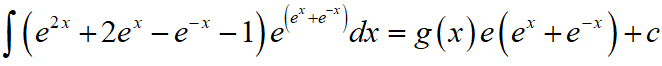where c is a constant of integration, then g(0) is

Options:

A. 1

B. e

C. e2

D. 2

Answer Key:

Solution:

JEE Main 2020 Maths Questions Answer Keys Solutions 5th Sep Shift 1 : Ques No 9

If α is the positive root of the equation, p(x) = x2 – x – 2 = 0, then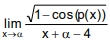is equal to

Options:

A. 3/2

B. 3/√2

C. 1/√2

D. 1/2

Answer Key:

Solution:

JEE Main 2020 Mathematics Questions with Answer Keys and Solutions : Ques No 10

If the co–ordinates of two points A and B are (√7, 0) and (-√7, 0) respectively and P is any point on
the conic, 9x2 + 16y2 = 144, then PA + PB is equal to :

Options:

A. 8

B. 16

C. 9

D. 6

Answer Key:

Solution:

JEE Main 2020 Maths Questions Answer Keys Solutions 5th Sep Shift 1 : Ques No 11

The product of the roots of the equation 9x2 – 18|x| + 5 = 0 is :

Options:

A. 25/81

B. 5/27

C. 25/9

D. 5/9

Answer Key:

Solution:

JEE Main 2020 Mathematics Questions with Answer Keys and Solutions : Ques No 12

If the volume of a parallelepiped, whose conterminous edges are given by the vectors a = i + j + nk, b = 2i + 4j – nk and c = i + nj + 3k (n > = 0) , is 158 cu-units, then :

Options:

A. n = 7

B. n = 9

C. b.c = 10

D. a.c = 17

Answer Key:

Solution:

JEE Main 2020 Maths Questions Answer Keys Solutions 5th Sep Shift 1 : Ques No 13

If the point P on the curve, 4x2 + 5y2 = 20 is farthest from the point Q(0, –4), then PQ2 is equal to :

Options:

A. 29

B. 48

C. 21

D. 36

Answer Key:

Solution:

JEE Main 2020 Mathematics Questions with Answer Keys and Solutions : Ques No 14

If (a,b,c) is the image of the point (1, 2, –3) in the linethen a + b + c is equal to :

Options:

A. n = 7

B. n = 9

C. b.c = 10

D. a.c = 17

Answer Key:

Solution:

JEE Main 2020 Maths Questions Answer Keys Solutions 5th Sep Shift 1 : Ques No 15

If the four complex numbers z, z, z – 2Re(z) and z – 2Re(z) represent the vertices of a square of side 4 units in the Argand plane, then |z| is equal to :

Options:

A. 4

B. 2

C. 4√2

D. 2√2

Answer Key:

Solution:

JEE Main 2020 Mathematics Questions with Answer Keys and Solutions : Ques No 16

If the minimum and the maximum values of the function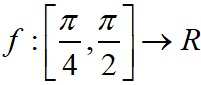defined byare m and M respectively, then the ordered pair (m, M) is equal to :

Options:

A. (–4, 4)

B. (0, 2√2)

C. (–4, 0)

D. (0, 4)

Answer Key:

Solution:

JEE Main 2020 Maths Questions Answer Keys Solutions 5th Sep Shift 1 : Ques No 17

The negation of the Boolean expression x ↔ ~ y is equivalent to :

Options:

A. (~ x ^ y) v (~ x ^ ~ y)

B. (x ^ y) v (~ x ^ ~ y)

C. (x ^ ~y) v (~ x ^ y)

D. (x ^ y) v (~ x v ~ y)

Answer Key:

Solution:

JEE Main 2020 Mathematics Questions with Answer Keys and Solutions : Ques No 18

If Let λ ∈ R. The system of linear equations 2x1 – 4x2 + λx3 = 1, x1 – 6x2 + x3 = 2 and λx1 – 10x2 + 4x3 = 3 is inconsistent for :

Options:

A. every value of λ

B. exactly two values of λ

C. exactly one positive value of λ

D. exactly one negative value of λ

Answer Key:

Solution:

JEE Main 2020 Maths Questions Answer Keys Solutions 5th Sep Shift 1 : Ques No 19

The mean and variance of 7 observations are 8 and 16, respectively. If five observation are  2, 4, 10, 12, 14 then the absolute difference of the remaining two observations is :

Options:

A. 1

B. 2

C. 3

D. 4

Answer Key:

Solution:

JEE Main 2020 Mathematics Questions with Answer Keys and Solutions : Ques No 20

The value of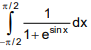is

Options:

A. π/4

B. π/2

C. 3π/2

D. π

Answer Key:

Solution:

Instructions for SECTION 2 : (Maximum Marks : 20):

This section contains FIVE (05) questions. The answer to each question is NUMERICAL VALUE with two digit integer and decimal upto one digit.

If the numerical value has more than two decimal places truncate/round-off the value upto TWO decimal places.

Full Marks : +4 If ONLY the correct option is chosen

Zero Marks : 0 In all other cases

JEE Main 2020 Maths Questions Answer Keys Solutions 5th Sep Shift 1 : Ques No 21

The number of words, with or without meaning, that can be formed by taking 4 letters at a time from the letters of the word ‘SYLLABUS’ such that two letters are distinct and two letters are alike, is _________.

Answer Key: 240.00

Solution:

JEE Main 2020 Mathematics Questions with Answer Keys and Solutions : Ques No 22

The natural number m, for which the coefficient of x in the binomial expansion of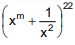is 1540, is

Answer Key: 13.00

Solution:

JEE Main 2020 Maths Questions Answer Keys Solutions 5th Sep Shift 1 : Ques No 23

If the line, 2x – y + 3 = 0 is at a distance 1/√5 and 2/√5 from the lines 4x – 2y + α = 0 and 6x – 3y + β = 0, respectively, then the sum of all possible values of α and β is_________

Answer Key: 30.00

Solution:

JEE Main 2020 Mathematics Questions with Answer Keys and Solutions : Ques No 24

Let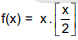for –10 < x < 10, where [t] denotes the greatest integer function. Then the number of points of discontinuity of f is equal to __________.

Answer Key: 08.00

Solution:

JEE Main 2020 Maths Questions Answer Keys Solutions 5th Sep Shift 1 : Ques No 25

Four fair dice are thrown independently 27 times. Then the expected number of times, at least two dice show up a three or a five, is __________.

Answer Key: 11.00

Solution:

error: Content is protected !!